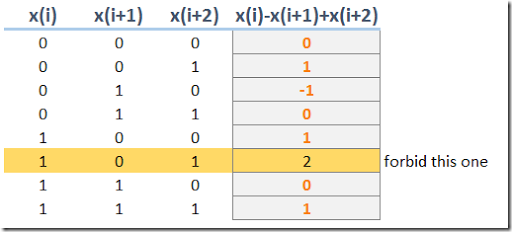Wednesday, April 5, 2017

MIP Modeling: preventing a bit-pattern

From (1):

Let us say we have $$n$$ binary variables $$x_i$$ for all $$i=1,2,…,n$$ i.e., $$x_i \in \{0,1\}$$ for all $$i=1,2,…,n$$.

I need to write the following constraint:

• If $$x_i=1$$ and $$x_{i+2}=1$$, then $$x_{i+1}=1$$. In other words, the variables $$x_i$$ must be consecutives.

Basically we want to prevent the sequence $$[1,0,1]$$ but allow all other patterns. This can be accomplished with the constraint:

 $\boxed{x_i-x_{i+1}+x_{i+2} \le 1}$

This is actually a special case of (2). Of course for this small example we can inspect all possible combinations (there are $$2^3=8$$ of them) to verify we do the right thing:Counting Start-ups

What about if you want to have all your $$x_i=1$$ consecutive. I.e. we only allow something like $$[0,0,1,1,1,1,0,0,0]$$ where there are no holes in the series of ones. This can be best modeled by counting the number of times we go from $$0$$ to $$1$$. A compact formulation is:

 \boxed{\begin{align}&s_i \ge x_i – x_{i-1}\\ &\sum_i s_i \le 1\\ &s_i \in \{0,1\}\end{align}}

Here we force $$s_i=1$$ if we go from $$0$$ to $$1$$. Note that we can relax $$s_i$$ to be continuous (i.e. $$s_i \in [0,1]$$).

This example would show:

 $\begin{array}{cccccccccc}x_i=&[0&0&1&1&1&1&0&0&0]\\ s_i=&[0&0&1&0&0&0&0&0&0]\end{array}$
References
1. How to model a consecutive binary constraint? http://math.stackexchange.com/questions/2218389/how-to-model-a-consecutive-binary-constraint
2. Integer Cuts, http://yetanothermathprogrammingconsultant.blogspot.com/2011/10/integer-cuts.html
3. Modeling number of machine start-ups, http://yetanothermathprogrammingconsultant.blogspot.com/2014/12/modeling-number-of-machine-start-ups.html